Virasoro algebra

(diff) ← Older revision | Latest revision (diff) | Newer revision → (diff)

A Lie algebra, denoted by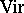, overwith basis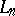(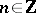),and the following commutation relations (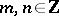):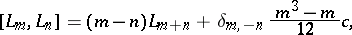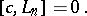Since the vector fields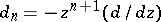() on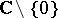satisfy the relation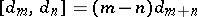, the Lie algebrais a central extension (which is, in fact, universal) of the Lie algebra of holomorphic vector fields on the punctured complex plane having finite Laurent series. For this reason the Virasoro algebra plays a key role in conformal field theory.

On the other hand, letting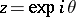, whereis the parameter on the unit circle, one gets. Hence the Lie algebra of vector fields on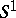with finite Fourier series is a real form of the Lie algebra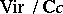consisting of elements fixed under the anti-linear involution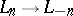,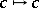. For this reason the Virasoro algebra is intimately related to the representation theory of the group of diffeomorphisms of, of the loop groups and to affine Kac–Moody algebras (see Kac–Moody algebra).

The representation theory of the Virasoro algebra has numerous applications in mathematics and theoretical physics. The most interesting, positive-energy representations ofin a complex vector space, are defined by the property thatacts as a scalar, denoted by the same letter(called the central charge), and that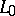(the energy operator) is diagonalizable with finite-dimensional eigenspaces and with real spectrum bounded below: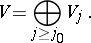The character of such a representation is the (formal) series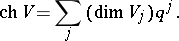The first positive-energy representations ofwere implicitly constructed by M.A. Virasoro [a1] in 1970, using an Abelian version of the Sugawara construction (see Kac–Moody algebra) in the framework of string theory. Since that time, and especially since the proof of the no-ghost theorem [a2], the representation theory of the Virasoro algebra has become a key ingredient of string theory (see [a3]). The Virasoro central extension itself was previously discovered by mathematicians [a4], [a5]; paper [a2] is one of the earliest references in the physics literature containing a correct formula for the central term.

An irreducible positive-energy representation ofin a vector spaceadmits a non-zero vector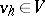, where, such that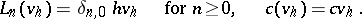(a1)

Then one has: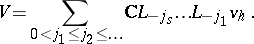(a2)

This representation is determined uniquely by the two real constants, the central charge, and, the conformal dimension, and is denoted by. It is called degenerate if (a2) is not a direct sum decomposition.

The first basic result of the representation theory of[a6] (see [a7] for a proof) states that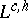is degenerate if and only if,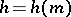for some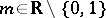and some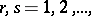whereThe basic idea of the foundational work [a8] on conformal field theory is to use degenerate representations ofto write down differential equations for correlation functions. The most complete results have been obtained [a9] for the "most degenerate" representations, called the minimal series representations. These correspond to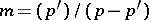, whereand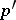are relatively prime positive integers and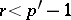,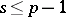[a8].

The characters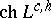were computed in [a10]. It follows that after letting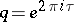, the functionbecomes a modular function inon the upper half-plane for someif and only ifis a representation of minimal series (then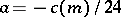) [a11].

The representationcarries a unique Hermitian form such that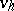has normand the operatorsand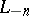are adjoint. Another important class of representationsare the unitary ones, i.e. those for which the Hermitian form is positive definite. The complete list of unitary representations is (see [a12], [a13], [a7]):

a),;

b) minimal series with.

The minimal series representations (especially the unitary ones) are intimately related to statistical lattice models (see [a14]). For example, the case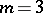is identified with the Ising model,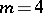with the Potts model, etc.

Among other areas of applications of the Virasoro algebra one should mention the theory of moduli spaces of curves (see [a15][a18]).